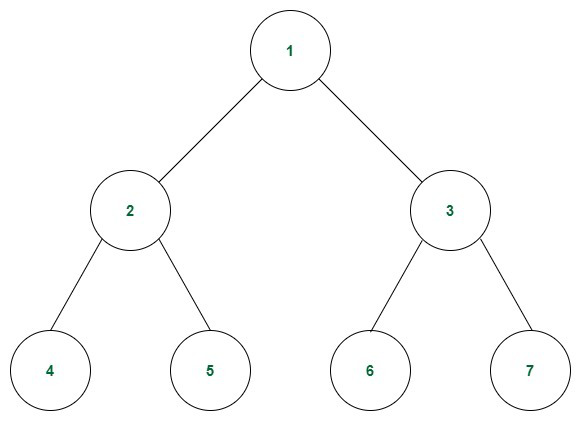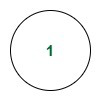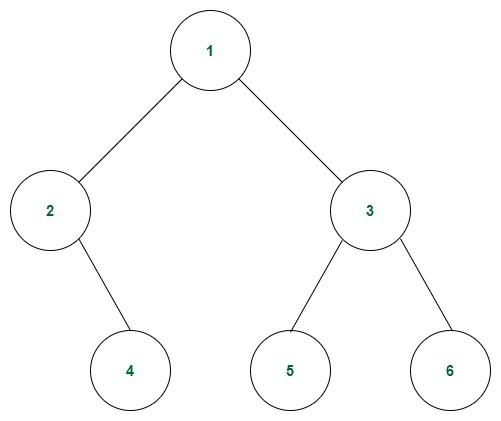GeeksforGeeks App
Open AppBrowser
Continue

## Related Articles

What is a Perfect Binary Tree?

A perfect binary tree is a special type of binary tree in which all the leaf nodes are at the same depth, and all non-leaf nodes have two children. In simple terms, this means that all leaf nodes are at the maximum depth of the tree, and the tree is completely filled with no gaps.

The maximum number of nodes in a perfect binary tree is given by the formula 2^(d+1) – 1, where d is the depth of the tree. This means that a perfect binary tree with a depth of n has 2^n leaf nodes and a total of 2^(n+1) – 1 nodes.

Perfect binary trees have a number of useful properties that make them useful in various applications. For example, they are often used in the implementation of heap data structures, as well as in the construction of threaded binary trees. They are also used in the implementation of algorithms such as heapsort and merge sort.

In other words, it can be said that each level of the tree is completely filled by the nodes.

Examples of Perfect Binary Tree:Example of a Perfect Binary Tree

A tree with only the root node is also a perfect binary tree.Example-2

The following tree is not a perfect binary tree because the last level of the tree is not completely filled.Not a Perfect Binary Tree

Properties of a Perfect Binary Tree:

• Degree: The degree of a node of a tree is defined as the number of children of that node. All the internal nodes have a degree of 2. The leaf nodes of a perfect binary tree have a degree of 0.
• Number of leaf nodes: If the height of the perfect binary tree is h, then the number of leaf nodes will be 2h because the last level is completely filled.
• Depth of a node: Average depth of a node in a perfect binary tree is Θ(ln(n)).
• Relation between leaf nodes and non-leaf nodes: No. of leaf nodes = No. of non-leaf nodes +1.
• Total number of nodes: A tree of height h has total nodes = 2h+1 – 1. Each node of the tree is filled. So total number of nodes can be calculated as 20 + 21 + . . . + 2h = 2h+1 – 1.
• Height of the tree: The height of a perfect binary tree with N number of nodes = log(N + 1) – 1 = Θ(ln(n)). This can be calculated using the relation shown while calculating the total number of nodes in a perfect binary tree.

Check whether a tree is a Perfect Binary Tree or not:

• Check the depth of the tree. A perfect binary tree is defined as a tree where all leaf nodes are at the same depth, and all non-leaf nodes have two children. To check whether a tree is a perfect binary tree, you can first calculate the depth of the tree.
• Check the number of nodes at each level: Once you have calculated the depth of the tree, you can then check the number of nodes at each level. In a perfect binary tree, the number of nodes at each level should be a power of 2 (e.g. 1, 2, 4, 8, etc.). If any level has a different number of nodes, the tree is not a perfect binary tree.